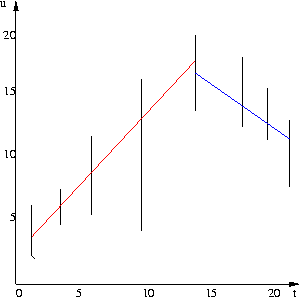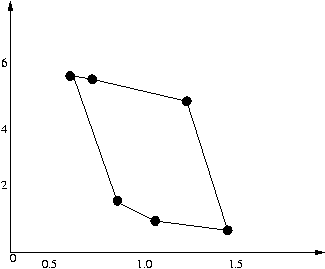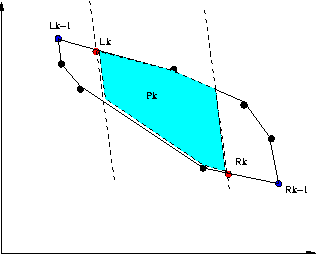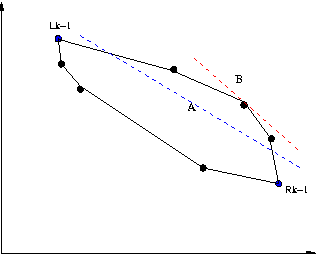# Line Fitting between Data Ranges

Contents

## 1. Introduction

In this project an online O(n) algorithm proposed by Joseph O'Rourke  for fitting straight lines to incremental data ranges is examined.

• Basic Idea

We consider data ranges [ak,wk] which are received at each time tk sequentially. Then, the set of all straight lines u = mt + b that fits the (tk, [ak,wk]), k= 1,2,...,n can be represented as the set of all (m,b) pairs that satisfy the equations

• ak < mtk + b < wk for k = 1,...n

(1)

•Figure 1. Example of t-b parameter space

• By changing our point of view from the u-t parameter space where the data live to the m-b space, we can consider the inequality (1) as the constraint equations of a linear programming problem of two variables m and b.

b > (-tk)m + ak
b < (-tk)m + wk

(2)
• Thus, the original problem of finding straight lines of n data ranges can be reduced to finding the intersection Pn of feasible regions Pk (or intersections of half-planes), k = 1,...,n constrained by the 2n parallel equations in (2).

•Figure 2. Example of m-b parameter space (representation of the first five data ranges of Figure 1.)

• Goal

Construct a convex polygon Pk from Pk-1 on-line in the m-b parameter space using the set of sequential inputs (tk, [ak,wk]).

## 2. Polygon Properties

Before we mention the algorithm it is necessary to go over the basic properties of polygons which are used in the algorithm. It is assumed that tk > 0 and tk < tk+1 for all k.

Lemma 1. Each edge of each polygon Pk in m-b parameter space has a strictly negative slope.

Proof. Polygon Pk is the intersection of 2k half-planes, and so each edge of Pk lies along one of the 2k half-plane edges. Each half-plane is described by one of the forms displayed as (2). Therefore, each plane edge has a slope of -ti. Since ti > 0 and ti < tk for 1 < i < k by the assumption, each slope lies within [-tk, 0].

Definition 1. Define mmax, bmax, mmin and bmin as the following.

mmaxk=max{m:(m,b) is a vertex of Pk}
bmaxk=max{b:(m,b) is a vertex of Pk}

mmink=min{m:(m,b) is a vertex of Pk}
bmink=min{b:(m,b) is a vertex of Pk}

Lemma 2. For each polygon Pk in m-b parameter space, the rightmost point of the polygon is also the lowest point, and the leftmost point is also the highest point.

Proof. Suppose that the rightmost and the lowest points are distinct. Then there exist m' and b' such that m' < mmaxk and b' > bmink and (mmaxk, b') and (m', bmink) are both vertices of Pk. It is not possible to close off Pk by connecting the rightmost and lowest vertices without adding at least one edge of positive slope. This contradicts Lemma 1. The assumption of the leftmost and highest points being distinct gives rise to the same contradiction.

Definition 2.The leftmost point Lk is defined by (mmaxk,bmaxk) and rightmost point Rk is defined by (mmink,bmink).

Definition 3. The upper half-polygonal chain Cu is defined by {vk: vk is a vertex of Pk which lies between Rk and Lk counter-clockwise order}. The lower half-polygonal chain Cl is defined by {vk: vk is a vertex of Pk which lies between Rk and Lk clockwise order}.

## 3. Algorithm

Input: (tk, ak, wk), i=1,...,n
Computed: Polygons P1, P2,...,Pn
Step 1. Compute P2 and initialize L2 and R2
Step 2. Repeat from k=3 to n

Step 2-1. If Rk-1 is to the left of the half-plane b > (-tk)m + ak or Lk-1 is to the right of the half-plane b < (-tk)m + wk then there is no straight line fitting data range at tk. Thus, reinitialize the process by setting Pk to the quadrilateral formed from the data ranges at tk-1 and tk. Initialize Lk and Rk.

Step 2-2. Else
Step 2-2-1. Search for the two intersection points of the half-plane b < (-tk)m + ak with Pk-1
(a) If Rk-1 is inside of the half-plane, go to Step 2-2-2.
(b) Find intersection point with edges of Cu counter-clockwisely.
(c) Find intersection point with edges of Cl clockwisely.
(d) Set the lowest intersection point as Rk

Step 2-2-2. Search for the two intersection points of the half-plane b > (-tk)m + wk with Pk-1
(a) If Lk-1 is inside of the half-plane, go to Step 2-2-3.
(b) Find intersection point with edges of Cu clockwisely.
(c) Find intersection point with edges of Cl counter-clockwisely.
(d) Set the highest intersection point as Lk
Step 2-2-3. Remove all vertices outside of the two intersection half-planes from Pk-1. Add in the new intersecting vertices.Figure 3. Example of constructing Pk from Pk-1 on-line

## 4. Correctness of Algorithm

To show that the algorithm computes Pk intersections correctly, we need to show the following.

(1) Each half-plane edge either does not intersect the polygon at all or intersects Cu once and Cl once.
(2)The algorithm correctly computes the intersection points.

The proof of (2) follows if (1) is proved to be true because of the design of the algorithm.

Proof of (1). From Lemma 1, all the edges of Pk-1 have slopes in the range [-tk-1, 0). Since tk > tk-1, the half-plane edge is steeper than any of the polygon edges. This implies that the half-plane edge intersects Cu and Cl exactly once and it enters the polygon if the intersection points are not Lk-1 or Rk-1. Figure 4 illustrates two impossible cases.Figure 4. Impossible cases of intersecting the half-plane and Pk-1

## 6. References

 O'Rourke, J., An On-Line Algorithm for Fitting Straight Lines Between Data Ranges, Comm. ACM, 24, 9, 574-578, 1981.

 Freedman, A.M., Buneman, O.P., Peckham, G., and Trattner, A., Automatic Recognition of Significant Events in the Vital Signs of Neonatal Infants. Compu. Biomed. Res. 12, 2, 141-148, 1979.

 Lee, D.T. and Preparata, F.P., An Optimal Algorithm for Finding the Kernel of a Polygon. J. ACM 26, 3, 415-421, 1979.

 O'Rourke, J., and Badier, N., Model-based Image Analysis of Human and Motion using Constraint Propagation, IEEE Trans. on Pattern Analysis and Machine Intelligence, PAMI-2, 6, 522-536, 1980.

 Pavlidis, T., Structural Pattern Recognition. Springer-Verlag, Berlin, 168-184, 1977.

 Preparata, F.P., An Optimal Real-time Algorithm for Plannar Convex Hulls. Comm. ACM, 22,7, 402-405, 1979.

 Shamos, M.I., and Hoey, D., Geometric Intersection Problems, 17th Ann. IEEE Symp. Foundations of Computer Science, 208-215, 1976.

 Shamos, M.I., Computational Geometry, PhD Dissertation, Yale University, 1978.